2022: SklogWiki celebrates 15 years on-line

# Entropy

"Energy has to do with possibilities. Entropy has to do with the probabilities of those possibilities happening. It takes energy and performs a further epistemological step." Constantino Tsallis 

Entropy was first described by Rudolf Julius Emanuel Clausius in 1865 . The statistical mechanical desciption is due to Ludwig Eduard Boltzmann (Ref. ?). The word entropy originated from the Greek word meaning a turning or transformation "τροπή" .

## Classical thermodynamics

In classical thermodynamics one has the entropy,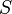$S$,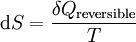${\mathrm d} S = \frac{\delta Q_{\mathrm {reversible}}} {T}$

where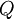$Q$ is the heat and$T$ is the temperature.

## Statistical mechanics

In statistical mechanics entropy is defined by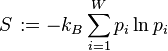$\left. S \right. := -k_B \sum_{i=1}^W p_i \ln p_i$

where$k_B$ is the Boltzmann constant,$i$ is the index for the microstates, and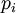$p_i$ is the probability that microstate i is occupied. In the microcanonical ensemble this gives: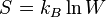$\left.S\right. = k_B \ln W$

where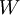$W$ (sometimes written as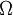$\Omega$) is the number of microscopic configurations that result in the observed macroscopic description of the thermodynamic system. This equation provides a link between classical thermodynamics and statistical mechanics

## Tsallis entropy

Tsallis (or non-additive) entropy  is defined as (Eq. 1)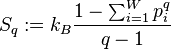$S_q:= k_B \frac{1-\sum_{i=1}^W p_i^q}{q-1}$

where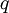$q$ is the Tsallis index . As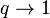$q \rightarrow 1$ one recovers the standard expression for entropy. This expression for the entropy is the cornerstone of non-extensive thermodynamics.

## Arrow of time

Articles:

Books:

• Steven F. Savitt (Ed.) "Time's Arrows Today: Recent Physical and Philosophical Work on the Direction of Time", Cambridge University Press (1997) ISBN 0521599458
• Michael C. Mackey "Time's Arrow: The Origins of Thermodynamic Behavior" (1992) ISBN 0486432432
• Huw Price "Time's Arrow and Archimedes' Point New Directions for the Physics of Time" Oxford University Press (1997) ISBN 978-0-19-511798-1